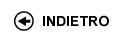Politecnico di Torino
01NLGJM, 01NLGLI, 01NLGLX, 01NLGMQ
Fundamentals of Engineering Thermodynamics and Heat Transfer
Corso di Laurea in Ingegneria Meccanica (Mechanical Engineering) - Torino
Corso di Laurea in Ingegneria Dell'Autoveicolo (Automotive Engineering) - Torino
Corso di Laurea in Ingegneria Elettrica - Torino
Espandi...
 Docente Qualifica Settore Lez Es Lab Tut Anni incarico Campagnoli ElenaRC ING-IND/10 53 27 0 0 12
 SSD CFU Attivita' formative Ambiti disciplinari ING-IND/10 8 C - Affini o integrative Attivitŕ formative affini o integrative
Esclusioni:
05IHQ
 Presentazione Understand: (i) The connections between the processes of the material systems and the energy exchanges. (ii) The fundamentals of elementary thermodynamics based on the first and the second law. (iii) How the theory of applied thermodynamics can be used to study energy conversions in power, propulsion and industrial processes. (iv) The fundamentals of the transfer of mechanical and thermal energy and radiation in solid and fluid systems applied to real systems, as heat exchangers Risultati di apprendimento attesi Be able to: (i) Use the theory of applied thermodynamics to calculate the energy balance of closed and open systems, in steady state and transient processes. (ii) Recognize the principal gas and steam power systems and calculate in the ideal case energy transfers and efficiency. (iii) Know, from a phenomenological point of view the principle types of heat transfer processes, conduction, convection and radiation, and evaluate the quantitative connection between heat fluxes and properties of physical materials. (iv) Evaluate how heat transfer occurs in real devices as heat exchangers. Prerequisiti / Conoscenze pregresse Calculus. Linear algebra. Fundamentals of Differential Equations. Fundamentals of Physics I and II. Programma THERMODYNAMICS: Scope of the thermodynamics. The main concepts and physical quantities, systems, states, state equations, processes, temperature, work and heat. Homogeneous systems and ideal gases. The principle of mechanical energy conservation and Bernoulli law. First and second law for closed and open systems. Total and internal energy. Enthalpy. Entropy and main kinds of irreversibility. The Carnot theorem Absolute temperature. Ideal gas cycles with and without regeneration (Otto, Diesel, Joule-Brayton and Stirling). Vapors and their properties, the Mollier's diagram, the ideal and actual vapor cycles, the regeneration practice, combined cycles. Joule-Thomson effect. Real gases. Refrigeration and heat pumps cycles. Ideal gas mixture and psychrometry. Moist air properties and psychrometric diagrams. Moist air processes and air conditioning. HEAT TRANSFER Phenomenological descriptions of thermal energy transport. Thermal conduction, Fourier's law and thermal conductivity. Heat transfer in one dimensional bodies by using both Cartesian and cylindrical coordinates. Electrical analogy and thermal resistance. Heat transfer in extended surface bodies. Fluid properties, viscosity, laminar and turbulent conditions, Reynolds number, velocity and temperature boundary layer. The Newton's law. Forced and natural convection. Dimensional analysis and main correlations Heat transfer by radiation: The black body, Stefan-Boltzmann's and Wien's law. Gray bodies and emissivity. Radiation heat transfer among black bodies and among grey bodies; electrical analogy. Heat exchangers types. Heat flow and temperature distributions in parallel and counter flow heat exchangers. Methods for calculation of real heat exchanger: F coefficient, LMTS (logarithmic mean temperature difference) and efficiency. Organizzazione dell'insegnamento The course is organized in theoretical and applied lectures( to learn to solve exercises that apply the subjects dealt with in lessons) and laboratory experiments. Testi richiesti o raccomandati: letture, dispense, altro materiale didattico - M. W. Zemansky, M.M. Abbott, H.C. Van Ness, "Basic engineering thermodynamics", Mc Graw Hill - M.J. Moran, H.N. Shapiro, 'Fundamentals of engineering Thermodynamics', J. Wiley & Sons, Inc., 2006. - P.S. Schmidt, O.A. Ezekoye, J.R. Howell, D.K. Baker, 'Thermodynamics: An Integrated Learning System', J. Wiley & Sons, Inc., 2006. - F.P. Incropera, D.P. De Witt, 'Fundamentals of Heat and Mass Transfer', J. Wiley & Sons, Inc - Y. A. Çengel, 'Introduction to thermodynamics and heat transfer", 2nd Edition, McGraw-Hill, 2008. - M.J. Moran, H.N. Shapiro, B.R. Munson, D.P. DeWitt, 'Introduction to Thermal Systems Engineering, Thermodynamics, Fluid Mechanics and Heat Transfer', J. Wiley & Sons, Inc., 2003. Criteri, regole e procedure per l'esame Written test, concerning the solution of some exercises, and oral on the main topics of the course. Orario delle lezioni Statistiche superamento esami Programma definitivo per l'A.A.2012/13